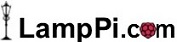Home Hardware Projects Code Notes

Notes

 Single-Dimension vs Multi-Dimension Arrays This should allow you to distinguish between single and multi-valued arrays ``` # Initialisation \$myArray = array(); \$array_count = count(\$myArray ); \$myValue = ""; # Perform some action \$multidimensional_array = (\$array_count <> count(\$myArray, 1)); if (\$multidimensional_array) { \$myValue = \$myArray[\$i][\$j]; }else{ \$myValue = \$myArray[\$i]; } ``` Note: Using "\$myValue = \$myArray[\$i][\$j];" on a single-value array can return unexpected results.